## LCM and HCF

#### LCM and HCF

1. The smallest number, which when increased by 5 is divisible by each of 24,32, 36 and 564, is

1. Required number = (LCM of 24, 32, 36 and 54) – 5
Now,LCM of 24, 32, 36 and 54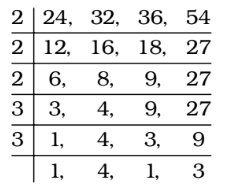LCM = 2 × 2 × 2 × 3 × 3 × 3 × 4 = 864

##### Correct Option: B

Required number = (LCM of 24, 32, 36 and 54) – 5
Now,LCM of 24, 32, 36 and 54LCM = 2 × 2 × 2 × 3 × 3 × 3 × 4 = 864
∴ Required number = 864 – 5 = 859

1. The number nearest to 43582 divisible by each of 25, 50 and 75 is :

1. LCM of 25, 50 and 75 = 150
On dividing 43582 by 150, remainder = 82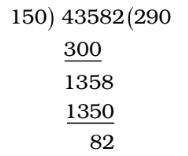∴ Required number = 43582 + (150 – 82)

##### Correct Option: B

LCM of 25, 50 and 75 = 150
On dividing 43582 by 150, remainder = 82∴ Required number = 43582 + (150 – 82) = 43650

1. The least number, which is a perfect square and is divisible by each of the numbers 16, 20 and 24, is

1. The smallest number divisible by 16, 20 and 24
= LCM of 16, 20 and 24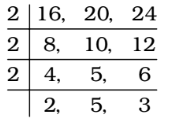∴ LCM = 2 × 2 × 2 × 2 × 5 × 3
LCM = 22 × 22 × 5 × 3

##### Correct Option: B

The smallest number divisible by 16, 20 and 24
= LCM of 16, 20 and 24∴ LCM = 2 × 2 × 2 × 2 × 5 × 3
LCM = 22 × 22 × 5 × 3
∴ Required complete square number = 22 × 22 × 52 × 32 = 3600

1. The largest 4-digit number exactly divisible by each of 12, 15, 18 and 27 is

1. As we know that Greatest n digit number which when divided by three numbers p,q,r leaves no remainder will be Required Number = (n – digit greatest number) – R , R is the remainder obtained on dividing greatest n digit number by L.C.M of p.q,r.
The largest number of 4-digits is 9999. L.C.M. of divisors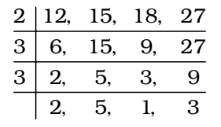LCM = 2 × 2 × 3 × 3 × 3 × 5 = 540

##### Correct Option: B

As we know that Greatest n digit number which when divided by three numbers p,q,r leaves no remainder will be Required Number = (n – digit greatest number) – R , R is the remainder obtained on dividing greatest n digit number by L.C.M of p.q,r.
The largest number of 4-digits is 9999. L.C.M. of divisorsLCM = 2 × 2 × 3 × 3 × 3 × 5 = 540
Divide 9999 by 540, now we get 279 as remainder.
9999 – 279 = 9720
Hence, 9720 is the largest 4-digit number exactly divisible by each of 12, 15, 18 and 27.

1. The number nearest to 10000, which is exactly divisible by each of 3, 4, 5, 6, 7 and 8, is :

1. LCM of 3, 4, 5, 6, 7, 8 = 840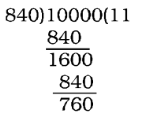Since, the remainder 760 is more than half of the divisor 840.

##### Correct Option: B

LCM of 3, 4, 5, 6, 7, 8 = 840Since, the remainder 760 is more than half of the divisor 840.
∴ The nearest number = 10000 + (840 – 760) = 10080